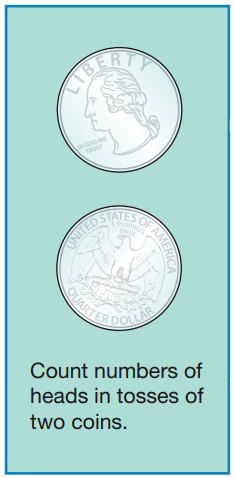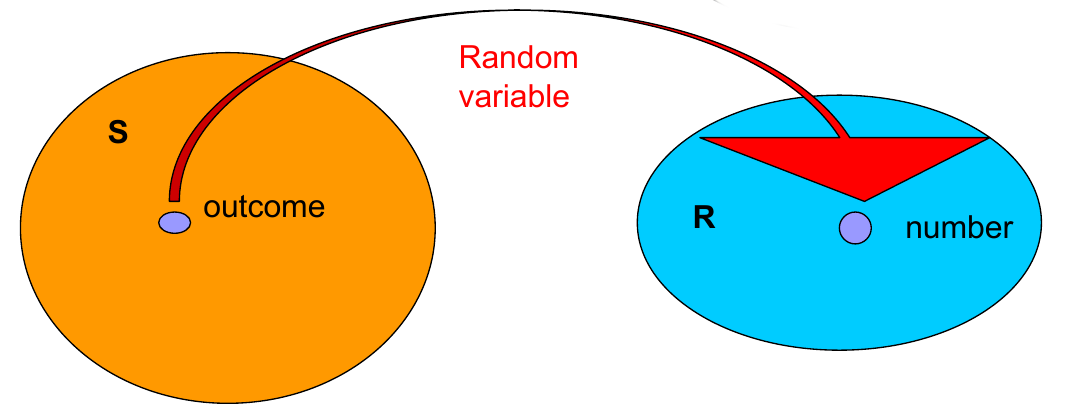# Probability Distributions, Mass Functions and Cumulative Distribution Functions

02/17/2021

## Instructions:

Use the left and right arrow keys to navigate the presentation forward and backward respectively. You can also use the arrows at the bottom right of the screen to navigate with a mouse.

FAIR USE ACT DISCLAIMER:
This site is for educational purposes only. This website may contain copyrighted material, the use of which has not been specifically authorized by the copyright holders. The material is made available on this website as a way to advance teaching, and copyright-protected materials are used to the extent necessary to make this class function in a distance learning environment. The Fair Use Copyright Disclaimer is under section 107 of the Copyright Act of 1976, allowance is made for “fair use” for purposes such as criticism, comment, news reporting, teaching, scholarship, education and research.

## Outline

• The following topics will be covered in this lecture:

• Random variables
• Probability distributions
• Probability mass functions
• Cumulative distribution functions

## Random VariablesCourtesy of Mario Triola, Essentials of Statistics, 6th edition

• Let us recall the idea of a random variable.
• Prototypically, we can consider the coin flipping example from the motivation:
• $$X$$ is the number heads in two coin flips.
• Every time we repeat two coin flips $$X$$ can take a different value due to many possible factors:
• how much force we apply in the flip;
• air pressure;
• wind speed;
• etc…
• The result is so sensitive to these factors that are beyond our ability to control, we consider the result to be by chance.
• Before we flip the coin twice, the value of $$X$$ has yet-to-be determined.
• After we flip the coin twice, the value of $$X$$ is fixed and possibly known.
• Formally we will define:
• Random Variable
A random variable is a function that assigns a real number to each outcome in the sample space of a random experiment.
• Notation
A random variable is denoted by an uppercase letter such as $$X$$. After an experiment is conducted, the measured value of the random variable is denoted by a lowercase letter such as $$x$$

### Random variables continuedCourtesy of Ania Panorska CC

• Suppose we are considering our sample space $$\mathbf{S}$$ of all possible outcomes of a random process.
• Then for any particular outcome of the process,
• e.g., for the coin flips one outcome is $$\{H,H\}$$,
• mathematically the random variable $$X$$ takes the outcome to the numerical value $$x=2$$ in the range $$\mathbf{R}$$.
• Note: $$X$$ must always take a numerical value.
• Because a random variable takes a numerical value (not categorical), we must consider the units that $$X$$ takes:
• Discrete random variable is a random variable with a finite (or countably infinite) range.
• In particular, the unit of $$X$$ cannot be arbitrarily sub-divided.